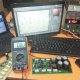Breaking News
Home / how to make inverter / how to make inverter 12v to 220v unlimited Watts

# how to make inverter 12v to 220v unlimited Watts

## how to make inverter 12v to 220v unlimited Watts:

Why am I telling unlimited watts? here in this, we are going to talk about easy inverter circuit diagram. we are not going to use any Ic or hard diagram. I think it will be so easy for you to make this inverter circuit diagram. let’s go to discuss this easy circuit diagram. let’s see that how to make inverter 12v to 220v unlimited Watts.

what do we need to make this inverter?

Transformer:

At first, we need a transformer. which transformer will be the step-up transformer. or we also can use step down transformer. if step-up transformer, the output will be 220 or 110 as we need. and input will be 12-0-12 voltage. here input means primary and output is secondary. or if we use normal transformer/step-down transformer. also, we can use. but for the step-down transformer, we have to use good quality one. that means a good amp one. minimum needs 10 amperes transformer. because our output watts depends on the transformer also.

Transistors:

Here in this inverter diagram, we use the 2n3055 transistor. We can call it a master transistor. because we can use this transistor in many places like power, inverter, sound etc. we can use various section in electronics fields.

Resistors:

In this diagram, we use the 330 ohms 5 watts resistor. we also can use 220 ohms 5 watts.

Let’s go to make the diagram, here we use 12 transistors for 200 Watts. if you want to more watts then you must need to add more transistors. it’s an easy diagram.

transistor circuit diagram of 2sa1943 and 2sc5200

Thanks a lot to be with us. If you want another post then please visit our website.

We have another post for you. like as repairing amplifier,